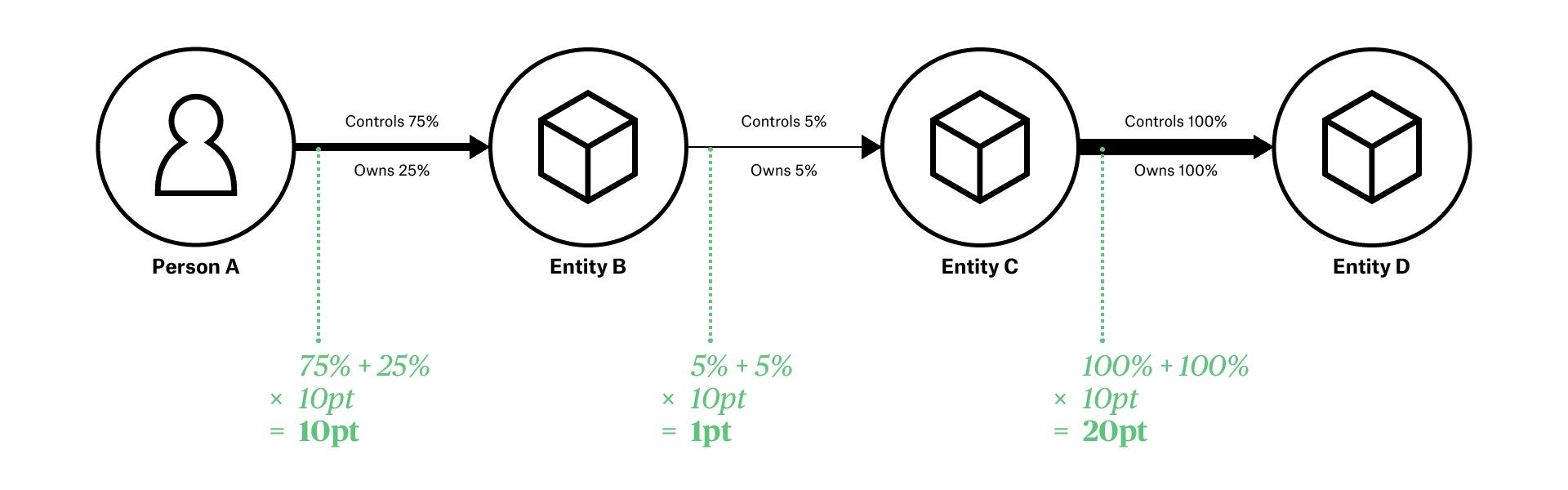# Beneficial Ownership Visualisation System

## Line Weight

Line Weight is an Optional Feature of BOVS Diagrams. It allows you to draw stronger relationships with heavier arrows, so that these stand out more.

The general rule is to use thicker Arrows for stronger relationships.### Unquantified Interests

If the interests in your diagram are not quantified, that is, you are not providing specific information about the strength of interest, you can use your subjective judgement to determine if some interests are stronger, and therefore should use a thicker Arrow.

If information about the strength of interest does in fact exist, you should prefer to include this information, and follow the approach below for quantified interests.

### Quantified Interests

If your diagram includes information about the strength of interests, then the weight of the Arrows should be drawn in proportion with the strength of interest.• Ideally, the Arrow thickness will be perfectly proportional to the strength of interest. For instance, if 10pt is your baseline, a 53% interest would be drawn using a 5.3pt Arrow.
• Where an Arrow represents two types of interest, i.e. both ownership and control, the weight of the Arrow would be the based on the sum of both types of interest. e.g. 60% ownership + 70% control gives a 130% Arrow (e.g. 13pt).
• A minimum weight should always be observed, such that Arrows representing low strengths of interest do not become invisible.
• Alternatively, the weight of the Arrow might correspond to ranges of interest, such as strengths of interest between 25-50%, 50-75%, etc.

#### Obscured Interests

If interests are obscured, use the following approaches to determine the weight of an Arrow:

• If the strength of interest is uncertain (is a range), draw the weight as if the value were the midpoint of the range.
• If the interest involves an unquantifiable property, use your subjective judgement to quantify the property, for the purposes of determining the Arrow’s weight only.
• For all other obscured interests, treat these as 50%.

### Colour

The Line Weight feature is best combined with the Optional Feature for Colour.